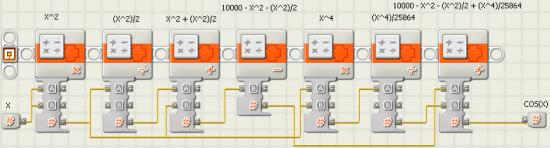# Computation of Cosine Function

If a high degree of accuracy is not required, and memory space is limited, then computation provides an alternative to table look up methods. The following example illustrates this approach for the cosine function.

Given a processor allowing 32 bit signed integer arithmetic, a fair approximation for cosine(x), scaled by a multiplier of 10000 is

```10000 * cos(x) = 10000 - X^2 - (X^2 / 2) + (X^4 / 25864)
```

where x is angle in degrees and "^" means exponentiation. For example, a^4 = aaaa. (This formula is derived from the Taylor series for the cosine function.)

The above formula may be implemented by NXT-G arithmetic blocks as shown belowThe above is done in the NXT-G programming environment. Each arithmetic block may be thought of as its NXT-G counter part, placed sequentially on the sequence beam.

The table below shows the values calculated by the above formula versus the more accurate, Excel cosine function.

Table 1
degreesapproximation
formula
Excel
function
% error
010000100000
5996399620
10985098480
15966496590
20940693970
25907890630
30868186600
35822181920
40769876600
45712170710
50649164281
55581657361
60510150001
65435342261
70357834202
75278625882
80198317362
8511818723
9038604